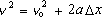This is one of the constant acceleration equations. It's the one with no "t" in it.

v is the velocity of the object at time t.
vo is the velocity of the object at time 0.
a is the acceleration.
Delta-x is x - xo. It is the displacement of the object from time 0 to time t.